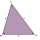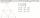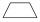# Line + trapezoid - math problems

#### Number of problems found: 18

• Diagonal intersectisosceles trapezoid ABCD with length bases | AB | = 6 cm, CD | = 4 cm is divided into 4 triangles by the diagonals intersecting at point S. How much of the area of the trapezoid are ABS and CDS triangles?
• ThereThere is a stretched steel cable between the 3 columns. The height of the first column is 4 m, the height of the second is 3.5 m. The distance between the first two columns is 2.5 m, the distance between the second and third is 5 m. The heels of all three
• Trapezium diagonalsIt is given trapezium ABCD with bases | AB | = 12 cm, |CD| = 8 cm. Point S is the intersection of the diagonals for which |AS| is 6 cm long. Calculate the length of the full diagonal AC.
• Diagonals at right angleIn the trapezoid ABCD, this is given: AB=12cm CD=4cm And diagonals crossed under a right angle. What is the area of this trapezoid ABCD?
• MO Z8–I–6 2018In the KLMN trapeze, KL has a 40 cm base and an MN of 16 cm. Point P lies on the KL line so that the NP segment divides the trapezoid into two parts with the same area. Find the length of the KP line.
• Trapezoid - intersection of diagonalsIn the ABCD trapezoid is AB = 8 cm long, trapezium height 6 cm, and distance of diagonals intersection from AB is 4 cm. Calculate the trapezoid area.
• MO Z9–I–2 - 2017In the VODY trapezoid, VO is a longer base and the diagonal intersection K divides the VD line in a 3:2 ratio. The area of the KOV triangle is 13.5 cm2. Find the area of the entire trapezoid.
• Trapezoid thirdsThe ABCD trapezoid with the parallel sides of the AB and the CD and the E point of the AB side. The segment DE divides the trapezoid into two parts with the same area. Find the length of the AE line segment.
• Rectangular trapezoidThe rectangular trapezoid ABCD is: /AB/ = /BC/ = /AC/. The length of the median is 6 cm. Calculate the circumference and area of a trapezoid.
• Trapezoid MO-5-Z8ABCD is a trapezoid that lime segment CE is divided into a triangle and parallelogram, as shown. Point F is the midpoint of CE, DF line passes through the center of the segment BE, and the area of the triangle CDE is 3 cm2. Determine the area of the trape
• Trapezium ABCDIn the figure, ABDC is a trapezium in which AB || CD. line segments RN and LM are drawn parallel to AB such that AJ=JK=KP. If AB=0.5m and AP=BQ=1.8m, find the lengths of AC, BD, RN and LM. angle D=angle C=60
• Internal anglesThe ABCD is an isosceles trapezoid, which holds: |AB| = 2 |BC| = 2 |CD| = 2 |DA|: On its side BC is a K point such that |BK| = 2 |KC|, on its side CD is the point L such that |CL| = 2 |LD|, and on its side DA the point M is such that | DM | = 2 |MA|. Dete
• Medians in triangleMedian of isosceles triangle has a length 3 cm. Determine the length of its sides if its perimeter is 16 cm.
• Isosceles trapeziumTrapezoid YUEB (YU||EB) is isosceles. The size of the angle at vertex U is 49 degrees. Calculate the size of the angle at vertex B.
• Draw a trapezoidDraw a trapezoid if given a = 7 cm, b = 4 cm, c = 3.5 cm, diagonal AC = 5cm. Solve as a construction task.
• Construction of trapezoidConstruct a trapezoid if b = 4cm, c = 7cm, d = 4,5cm, v = 3 cm (Procedure, discussion, sketch, analysis, construction)
• Inner anglesThe magnitude of the internal angle at the main vertex C of the isosceles triangle ABC is 72°. The line p, parallel to the base of this triangle, divides the triangle into a trapezoid and a smaller triangle. How big are the inner angles of the trapezoid?
• Divide an isosceles triangleHow to divide an isosceles triangle into two parts with equal contents perpendicular to the axis of symmetry (into a trapezoid and a triangle)?

Do you have an interesting mathematical word problem that you can't solve? Submit a math problem, and we can try to solve it.

We will send a solution to your e-mail address. Solved examples are also published here. Please enter the e-mail correctly and check whether you don't have a full mailbox.

Please do not submit problems from current active competitions such as Mathematical Olympiad, correspondence seminars etc...

Line - math problems. Trapezoid Problems.